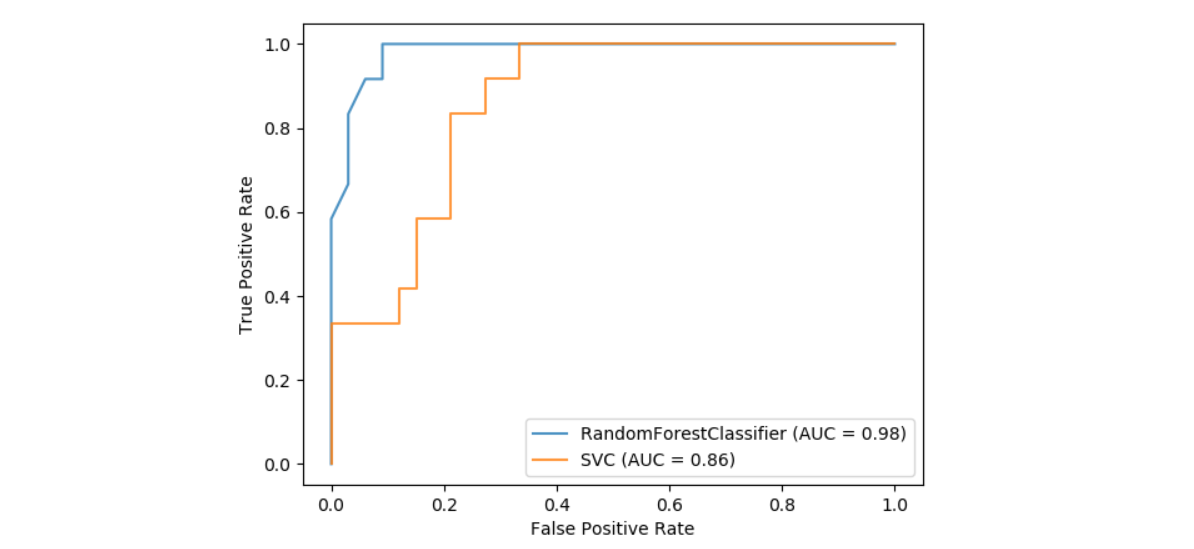# 带有可视化API的ROC曲线¶

Scikit-learn定义了一个简单的API，用于创建用于机器学习的可视化。 该API的主要功能是无需重新计算即可进行快速绘图和视觉调整。 在此示例中，我们将通过比较ROC曲线来演示如何使用可视化API。

import matplotlib.pyplot as pltfrom sklearn.svm import SVCfrom sklearn.ensemble import RandomForestClassifierfrom sklearn.metrics import plot_roc_curvefrom sklearn.datasets import load_winefrom sklearn.model_selection import train_test_splitX, y = load_wine(return_X_y=True)y = y == 2X_train, X_test, y_train, y_test = train_test_split(X, y, random_state=42)svc = SVC(random_state=42)svc.fit(X_train, y_train)

svc_disp = plot_roc_curve(svc, X_test, y_test)plt.show()

rfc = RandomForestClassifier(n_estimators=10, random_state=42)rfc.fit(X_train, y_train)ax = plt.gca()rfc_disp = plot_roc_curve(rfc, X_test, y_test, ax=ax, alpha=0.8)svc_disp.plot(ax=ax, alpha=0.8)plt.show()脚本的总运行时间：0分钟0.233秒